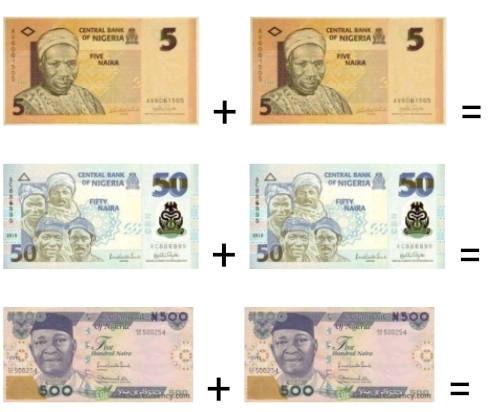# Lesson Notes By Weeks and Term - Nursery 2

Counting/identification of numbers 1 - 800

SUBJECT: Numeracy

TERM: 3rd Term

Week: 6

CLASS – Nursery 2

AGE – 5 years

DURATION – 5 periods of 35 minutes each

TOPIC- Counting/identification of numbers 1-800

CONTENT – Writing 500-550, Number endings. Number spellings 1-110. 10x table. Fractions. Money problems

INSTRUCTIONAL OBJECTIVES – By the end of the lesson, the pupils should be able to –

1. Count and identify numbers 1-800
2. Write numbers 500-550
3. Write in words 1-110
4. Recite the 10x table
5. Subtract fractions
6. Solve money problems

INSTRUCTIONAL MATERIALS – flash cards, charts, counters

INSTRUCTIONAL TECHNIQUES – Explanation, repetition, questions and answers

ENTRY BEHAVIOUR – The pupils can count numbers 1-750 and spell numbers 1-100

SET INDUCTION –She uses number rhyme to arouse their interest

INSTRUCTIONAL PROCEDURE

PERIOD I: Counting numbers 1-800 and writing 500-550

 STEPS TEACHER’S ACTIVITY PUPILS’ ACTIVITY IIntroduction The teacher reviews the previous lesson introduces the new topic Pupils participate IICounting Using the number chart, she counts number 1-800 repeatedly. Pupils repeat after her IIIWriting numbers She counts and write numbers 500-550 on the board; she also uses flashcards Pupils count and write in their book IVNumber ending She writes the number endings on the board and also uses flashcards Pupils count Evaluation Count and write numbers 500-550 Conclusion She marks their books and corrects them where necessary

PERIOD II& III: Number spellings 1-110. Addition of fractions, 10x times table

 STEPS TEACHER’S ACTIVITY PUPILS’ ACTIVITY IIntroduction The teacher reviews the previous lesson introduces the new topic Pupils participate IIDrilling She drills them on the spelling of 101-110One hundred and one = 101One hundred and two = 102One hundred and three = 103One hundred and four = 104One hundred and five = 105One hundred and six = 106One hundred and seven = 107One hundred and eight = 108One hundred and nine = 109One hundred and ten = 110 IIISubtraction of  fractions She draws some fractions on the board and explains how to subtract themFor example;Pupils identify heavy and light objects III10x table She writes the 10x table on the board and recites it severally as the pupils recite after her10 x 1 = 1010 x 2 = 2010 x 3 = 3010 x 4 = 4010 x 5 = 5010 x 6 = 6010 x 7 = 7010 x 8 = 8010 x 9 = 9010 x 10 = 10010 x 11 = 12010 x 12 = 140 Evaluation 1. Subtract these fractions2. Write in words 101-110101 =102 =103 =104 =105 =106 =107 =108 =109=110 =3. Complete the 10x table10 x 5 =10 x 6 =10 x 7=10 x 8= Conclusion She marks their books and corrects them where necessary

 STEPS TEACHER’S ACTIVITY PUPILS’ ACTIVITY IIntroduction The teacher reviews the basic shapes and introduces the new topic Pupils respond IIAddition of money The teacher displays all the currency notes and solves some addition of money problemsPupils pay attention Evaluation Add upConclusion She marks their books and corrects them where necessary